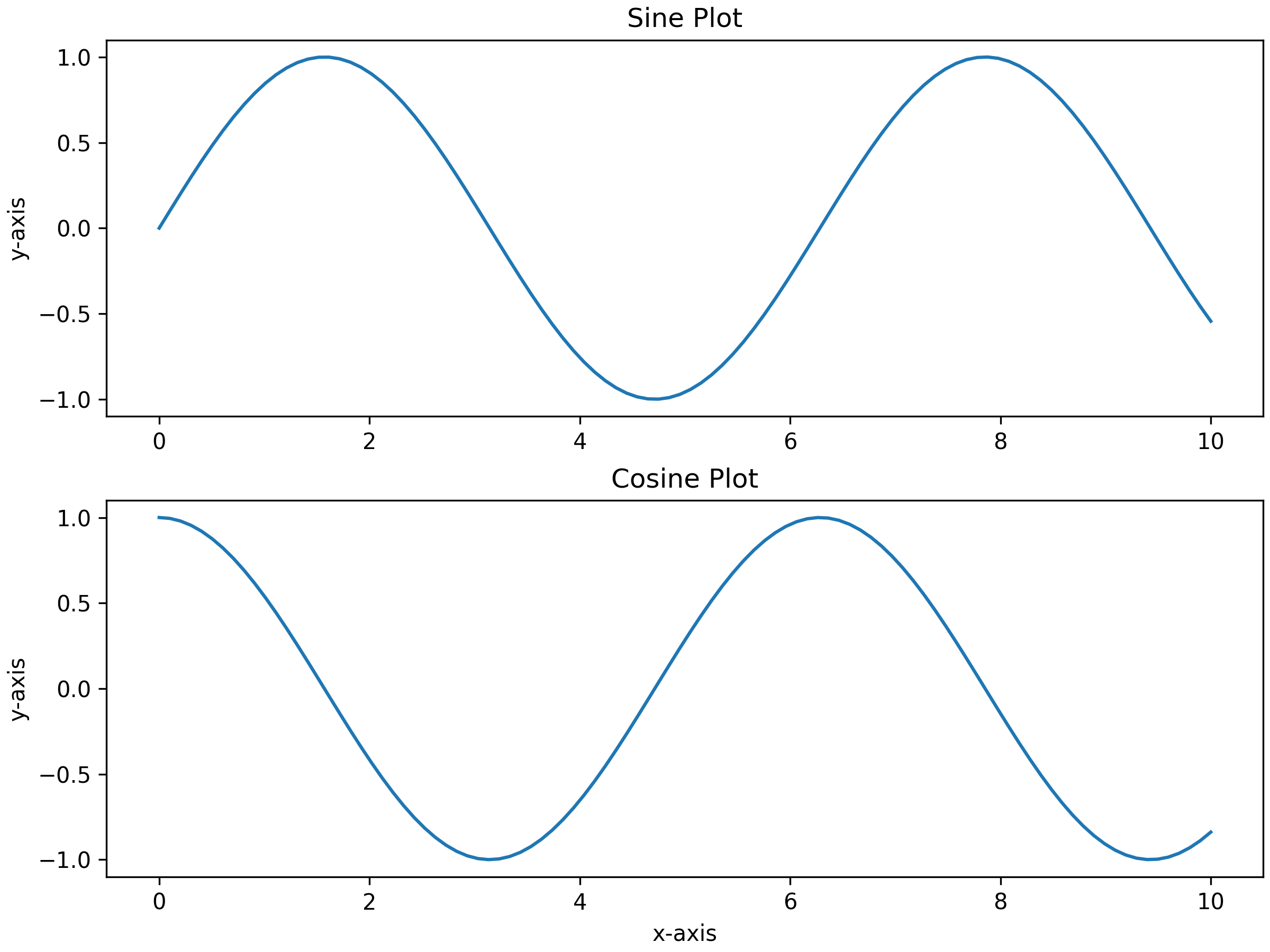# .subplots()

The `.subplots()` method returns a figure with multiple subplots. This method is an efficient way to create popular subplot layouts with a high-level and concise syntax.

## Syntax

``````pyplot.subplots(nrows, ncols)
``````

`.subplots()` has the following parameters:

Parameter Name Data Type(s) Usage
`nrows`, `ncols` int The quantity of rows and/or columns within the grid of subplots. Defaults to 1.
`sharex`, `sharey` bool or one of the following arguments: ‘none’, ‘all’, ‘row’, ‘col’ `sharex` and `sharey` control the sharing of properties among x or y axis of subplots. Shared x-axis or y-axis allows for creating tick labels only on the bottom subplot or first column subplot, respectively. Default is `False`.
`squeeze` bool Removes additional dimensions from the array of `Axes` when set to `True` (default). If set to `False`, it will always return a 2D array of `Axes` instances.
`width_ratios` input of length (`ncols`) Defines the proportional width of columns, each column width is given by width_ratios[i] / sum(width_ratios). By default, all columns have equal width.
`height_ratios` array-like input of length (`nrows`) Sets the proportional height of rows, dividing the total height among them. If not provided, all rows have an equal height.
`subplot_kw` dict Dictionary that includes keywords that are passed to the `add_subplot` method, which is used to generate each subplot.
`gridspec_kw` dict Dictionary that contains keywords passed to the `GridSpec` constructor for creating the grid where subplots are positioned.
`**fig_kw` Any other keyword arguments are passed to the `pyplot.figure` method.

## Example

The following example returns a `1` x `2` grid of `Axes` with a pair of sinusoidal plots.

```import matplotlib.pyplot as pltimport numpy as np
# Create some sample datax = np.linspace(0, 10, 100)y1 = np.sin(x)y2 = np.cos(x)
# Create a figure with two subplots, arranged verticallyfig, axs = plt.subplots(2, 1, figsize=(8, 6), layout="constrained")
# Plot the first subplot (top)axs.plot(x, y1)axs.set_title('Sine Plot')axs.set_ylabel('y-axis')
# Plot the second subplot (bottom)axs.plot(x, y2)axs.set_title('Cosine Plot')axs.set_xlabel('x-axis')axs.set_ylabel('y-axis')
# Display the figureplt.show()
```

The output is shown below: